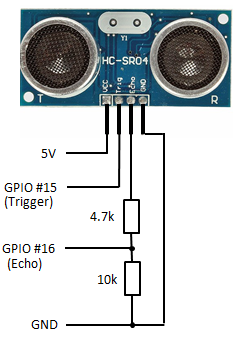Warning: include(highlight.inc.php): failed to open stream: No such file or directory in /usr/share/nginx/html/drucken.php on line 12

Warning: include(): Failed opening 'highlight.inc.php' for inclusion (include_path='.:/usr/local/lib/php') in /usr/share/nginx/html/drucken.php on line 12

## MISCELLANEOUS APPLICATIONS### Experiment 1: Use the HC-SR04 ultrasonic distance detector

Aim:
Measure the distance to a reflecting object by the travelling time of a ultrasonic pulse. Display the result in the output area. The A button is used to terminate the program.

The HC-SR04 module is simple to use. Apply a positive trigger pulse of about 10-100 us to the Trigger input. After a while the Echo output sends back a positive pulse whose length corresponds to the travel time of the ultrasonic signal. Below the oscilloscope image with the trigger and echo pulses.Since the speed of sound is 343 m/s, the sound travels 24 cm in 700 us. Because this is the distance from the sensor to the target and back to the sensor, the target is 12 cm away.

 The Echo output delivers a voltage around 5V, so a voltage divider with two resistors must be used to lower the voltage to the maximal accepted input voltage of a GPIO port (3.3V).Program:[►]

```# Ultrasonic1.py
# Using HC-SR04 ultrasonic module

import RPi.GPIO as GPIO
import time

P_TRIGGER = 15
P_ECHO = 16

def setup():
GPIO.setmode(GPIO.BOARD)
GPIO.setup(P_TRIGGER, GPIO.OUT)
GPIO.setup(P_ECHO, GPIO.IN)

def getDistance(timeoutCount  = 10000):
# Send max 10 us trigger pulse
GPIO.output(P_TRIGGER, GPIO.HIGH)
time.sleep(0.00001)
GPIO.output(P_TRIGGER, GPIO.LOW)
# Wait for HIGH signal
count1 = 0
while GPIO.input(P_ECHO) == GPIO.LOW and count1 < timeoutCount:
count1 += 1
startTime = time.time()
# Wait for LOW signal
count2 = 0
while GPIO.input(P_ECHO) == GPIO.HIGH and count2 < timeoutCount:
count2 += 1
if count1 == timeoutCount or count2  == timeoutCount:
return -1
elapsed = time.time() - startTime
distance =  34300 * elapsed / 2.0
# round to 2 decimals
distance = int(distance * 100 + 0.5) / 100.0
return distance

print "starting..."
setup()
while True:
d = getDistance()
print "d =", d
time.sleep(0.1)

```
Highlight program code (Ctrl+C copy, Ctrl+V paste)

In order to avoid a hanging program when the sensor does not reply, we use a timeout count and getDistance() returns -1.

You get a standalone application if you write out the result to an attached Oled or 7-segment display.

Program:[►]

```# Ultrasonic2.py
# Show distance on Oled

import RPi.GPIO as GPIO
import time
from OLED1306 import OLED1306

P_ESCAPE = 12 # Button A
P_TRIGGER = 15
P_ECHO = 16

def setup():
GPIO.setmode(GPIO.BOARD)
GPIO.setup(P_TRIGGER, GPIO.OUT)
GPIO.setup(P_ECHO, GPIO.IN)
GPIO.setup(P_ESCAPE, GPIO.IN)

def getDistance(timeoutCount  = 10000):
# Send max 10 us trigger pulse
GPIO.output(P_TRIGGER, GPIO.HIGH)
time.sleep(0.00001)
GPIO.output(P_TRIGGER, GPIO.LOW)
# Wait for HIGH signal
count1 = 0
while GPIO.input(P_ECHO) == GPIO.LOW and count1 < timeoutCount:
count1 += 1
startTime = time.time()
# Wait for LOW signal
count2 = 0
while GPIO.input(P_ECHO) == GPIO.HIGH and count2 < timeoutCount:
count2 += 1
if count1 == timeoutCount or count2  == timeoutCount:
return -1
elapsed = time.time() - startTime
distance =  34300 * elapsed / 2.0
# round to 2 decimals
distance = int(distance * 100 + 0.5) / 100.0
return distance

print "starting..."
oled = OLED1306()
oled.setFontSize(50)
setup()
while GPIO.input(P_ESCAPE) == GPIO.LOW:
d = getDistance()
oled.setText(str(d))
time.sleep(1)
GPIO.cleanup()
oled.setText("done")

```

Highlight program code (Ctrl+C copy, Ctrl+V paste)

### Experiment 2: Relay switching

Aim:
In many standard applications you want to control a device that is powered with an external power supply (up to 24V/10 A direct current) or the power line (up to 115-230V/10A alternating current). The good old relay using a solenoid coil to close/open contacts is used here.

Caution: Do not use the mains voltage unless you are familiar with high voltage. Be extremely careful to avoid an electric shock hazard. The relay should be part of a fully closed housing, e.g. a power outlet box. Avoid any electric contact between the high voltage and low voltage side.

Below an classical example circuit using a bipolar transistor that may be modified by using compatible parts. The diode parallel to the relay coil is needed to protect the transistor from voltage spikes caused by induction when the coil current is switched off.Remarks:
Instead of a solenoid relay, a solid-state such as the Crydon ASO242 (up to 2A @ 280V AC) can be used. Because of the required input voltage of 4-10V, a driver should be used, such as a bipolar transistor as above or the ULN2803. (A protecting diode is not needed.) The relay can be mounted in a power outlet using a screw terminal, as shown below.### Experiment 3: Step-up Converter 3.3V to 5 V

Aim:
In some systems only 3.3V is used for all electronics, so 5 V is not available to power 5 V electronics, motors or sensors (needed for many Arduino-based addons). Using a cheap voltage converter may resolve the problem. The same converters are used to generate 5V from a 3.6 V Lithium batteries.

 DC-DC Power Supply Converter Step Up Circuit 3V to 5V (from Ebay)